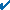# Puzzles

Our math puzzles hone students' vocabulary skills the fun way! We have interactive crossword puzzles with three levels of difficulty. Each crossword has a timer and buttons to help you. We also have interactive word search puzzles to keep you engaged. Our math puzzles are designed to supplement our in-depth units of instruction. Solutions to all puzzles are also provided. Choose a puzzle theme below to get started!

 Interactive Puzzles from Math Goodies Theme Crossword Word Search PolygonsCirclesElementary Math - Number TheoryIntroduction to FractionsAdd & Subtract Fractions & Mixed NumbersMultiply & Divide Fractions & Mixed NumbersAdding & Subtracting DecimalsMultiplying & Dividing DecimalsTheme Crossword Word Search Understanding PercentConsumer Math with Percent ApplicationsIntegersProbabilityPre-AlgebraRange, Mean, Median and ModeMathematical LogicData and GraphsSet Theory